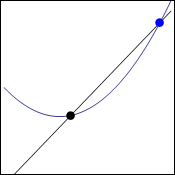# Quick point about equations and graphs

Hi,
mliae asked me to make some simpler post so she understands this post. So here it comes, she said that it was long since she used equations:

Well equation is something like this:

x=1

That is quite clear. Of course you can have very complicated equation with many “unknowns” which are usually noted as letters, x for example. All thats easy and it says that something (x) is equal to one, it has the value of 1.

We can manipulate these equations if we abide one rule: both sides (from left and right of equal sign) have to be manipulated. If we add one we have to do it on both sides:

x+1=1+1     —>    x+1=2

Easy. We can substitute in equations if we work with more:

a=b+42
2a+3=x

2(b+42)+3=x    (“a” was substitued by b+42 because that is what it equals to.)

Now in the post that is our concern we used this equation:To get here we have to use graph but I did not stop on that very much so I will go through it again.

Points on graph have two coordinates. This is because graph has 2 dimensions. These coordinates are usually called x and y and they are noted like this:

(x;y)

x says how much the point is to the left or right and y says how up or down (or closer/ further)

We had two points there on the graph:Now we will take the blue point as stacionary of course but since we are working in general and not with specific numbers, it should not matter.

So black point has coordinates x and y (x;y)

Since we want it to be general we will left it like this except the y. This is because we are going to derive functions and in those y=f(x) which means that y coordinate of the point is f(x). That is the notation that is used. We are working with the function f that gave coordinate y to our black point.

black_point(x;f(x))
blue_point(x+h;f(x+h))

The h should be clear from last time. It is the distance from the black point. Then when you insert this into the “slope” equation which I talked about here, you will get what you want.

Dragallur

Feel free to ask for more clarification.

# Limits made easy and heart equation

Hi,
I said that I started to learn limits. Since often I write about the things I just learn I will start this series today with the very basic. (The start is actually so easy you wont even think that it is precalculus or something)

Basicly the context for limits are functions. I have personally never learned functions onBasic idea of function

their own and I do plan to do that but I do not think it is so important right now. You just
have to have this idea that when you have function you will give it some input and it will give out some output (kind of black box).
It is important to say that one input corresponds only to one output. You have already probably seen some graphs so it is good to say that you can plot a function.

The thing with outputs and inputs can be nicely illustrated on one thing: imagine a class of kids and that you measure them.

Carl – 157 cm
Ann – 152 cm
Caroline – 160 cm
… and so on

Now you will plot them on graph next to each other. In big enough class you would probably have more kids with same height.On the left we have three example kids from one class. As is written, Ann has 152 centimeters, Susan 152 too but Jacob has 149,155 and 161 centimeters. Such class would not make a great function! The interesting thing is of course that to one height (152 cm) you can match more people (Ann and Susan), this makes sense. But you can not match one person to more heights! This is the way function works. You could just change names and heights for x and y and you would be there. So if you graph some function it will never be vertical and basicly no two values will ever be above each other, when you on the other hand plot equation you can easily get graph where there are two points above each other:Equation stolen from twitter and modified for better look.

So… what role do limits play here? As you probably already heard, limit tolds us what value will function give us when we give it input really close to some number. If you have function like

f(x)=x^2                  (f(x) is the way functions are noted, you could use other symbols)

You can ask for limit of x approaching to any real number and you would get the same as if you would calculate x to the power of 2. The real usage of limits comes when there is point where you can not get some nice value and also I think that it is defined by limits if function is continous (without jumps) or not.

I will slowly continue in the next post 😉

Dragallur

PS: some of my readers mathematicians (or anybody), if there please point out any mistakes, I am just learning this so it would be great to know my mistakes!

 To make it even clearer I made this extra picture: